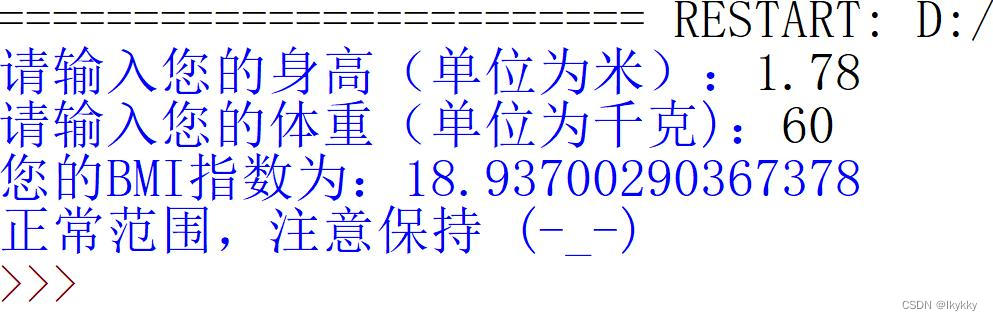### Python课程第二章实例训练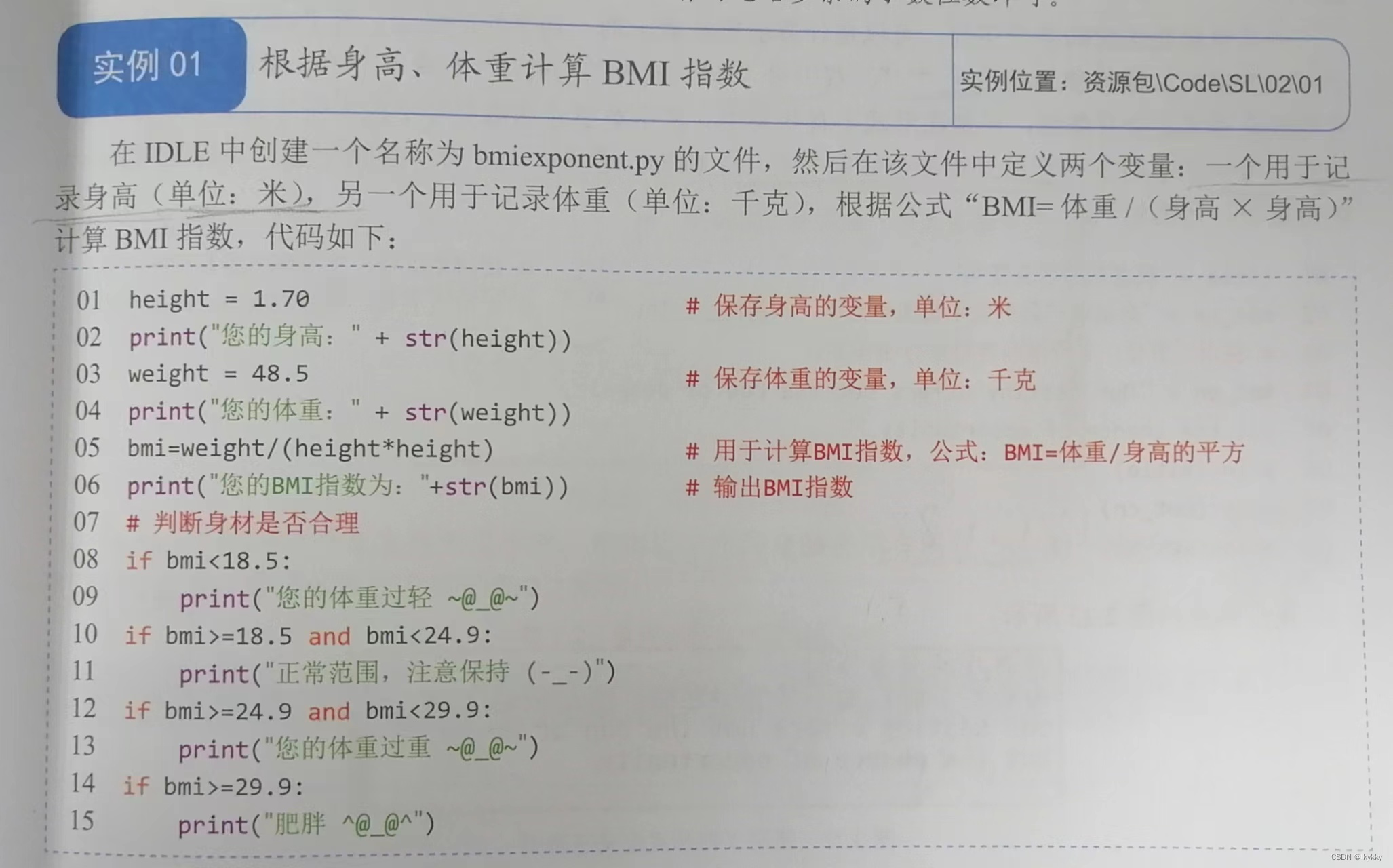``height=1.78print("您的身高："+str(height))weight=59.6print("您的体重："+str(weight))bmi=weight/(height*height)print("您的BMI指数为："+str(bmi))#判断身材是否合理if bmi<18.5:    print("您的体重过轻~@-@~")if bmi>=18.5 and bmi<24.9:    print("正常范围，注意保持（^!^）")if bmi>=24.9 and bmi<29.9:    print("您的体重过重>.<")if bmi>=29.9:    print("肥胖*。*")``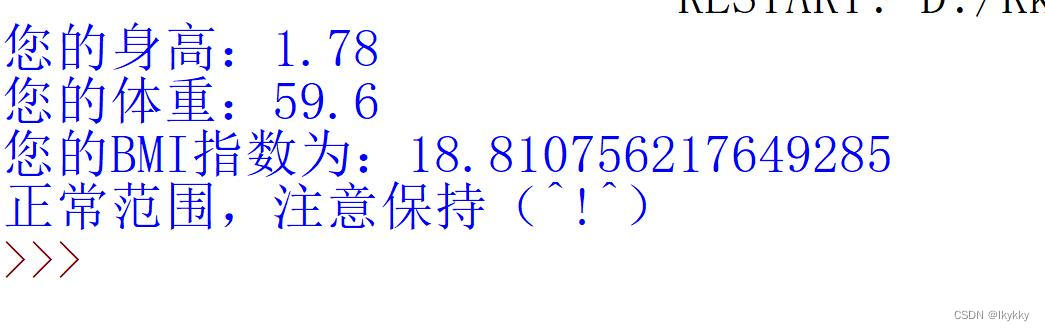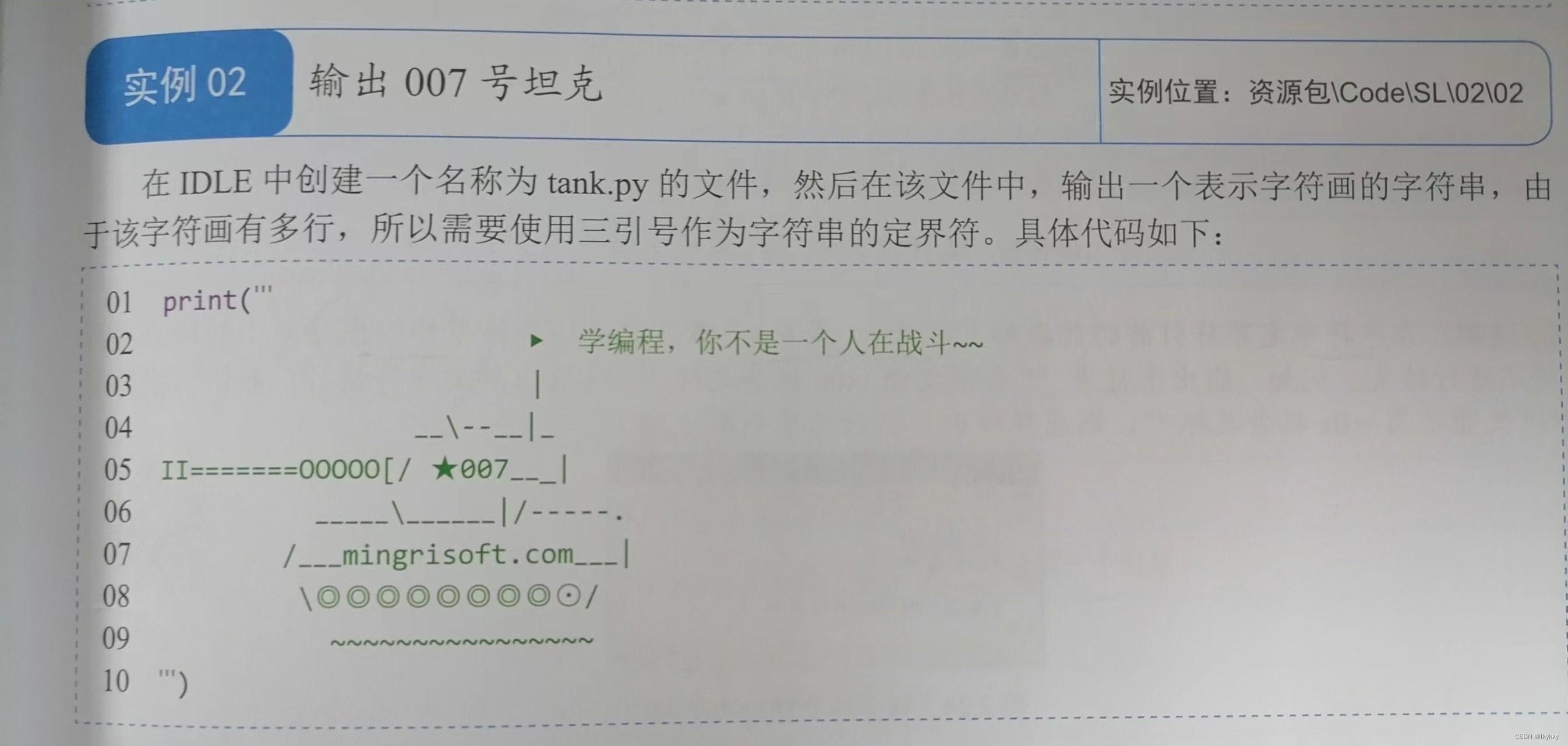``print('''                           ▶ 学编程，和许佳琪一直战斗~~~~                           |                        \--|     II========00000[[[[[[[]]]]]]                     \        /----、                /`````````````     |                 ▔▔越努力越幸运▔|                  \@@@@@@@@@@@@@@@/''')``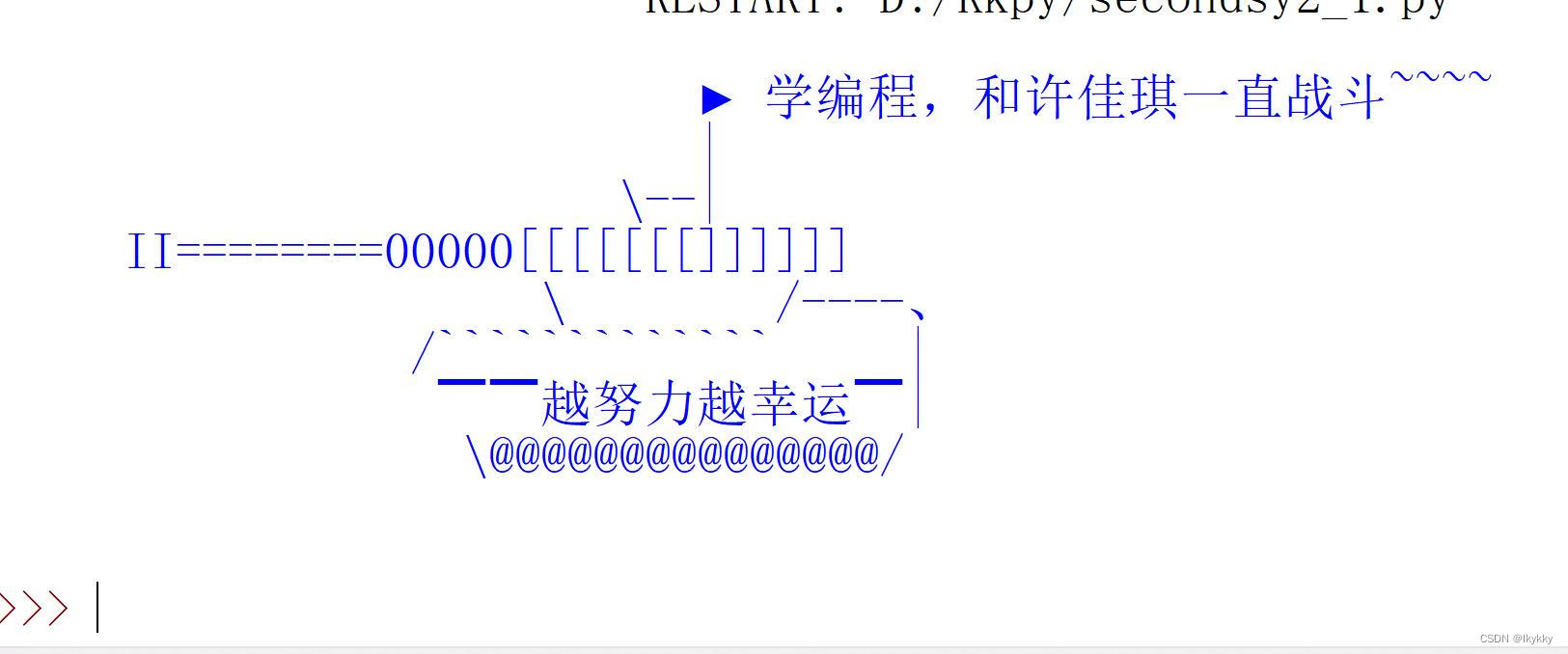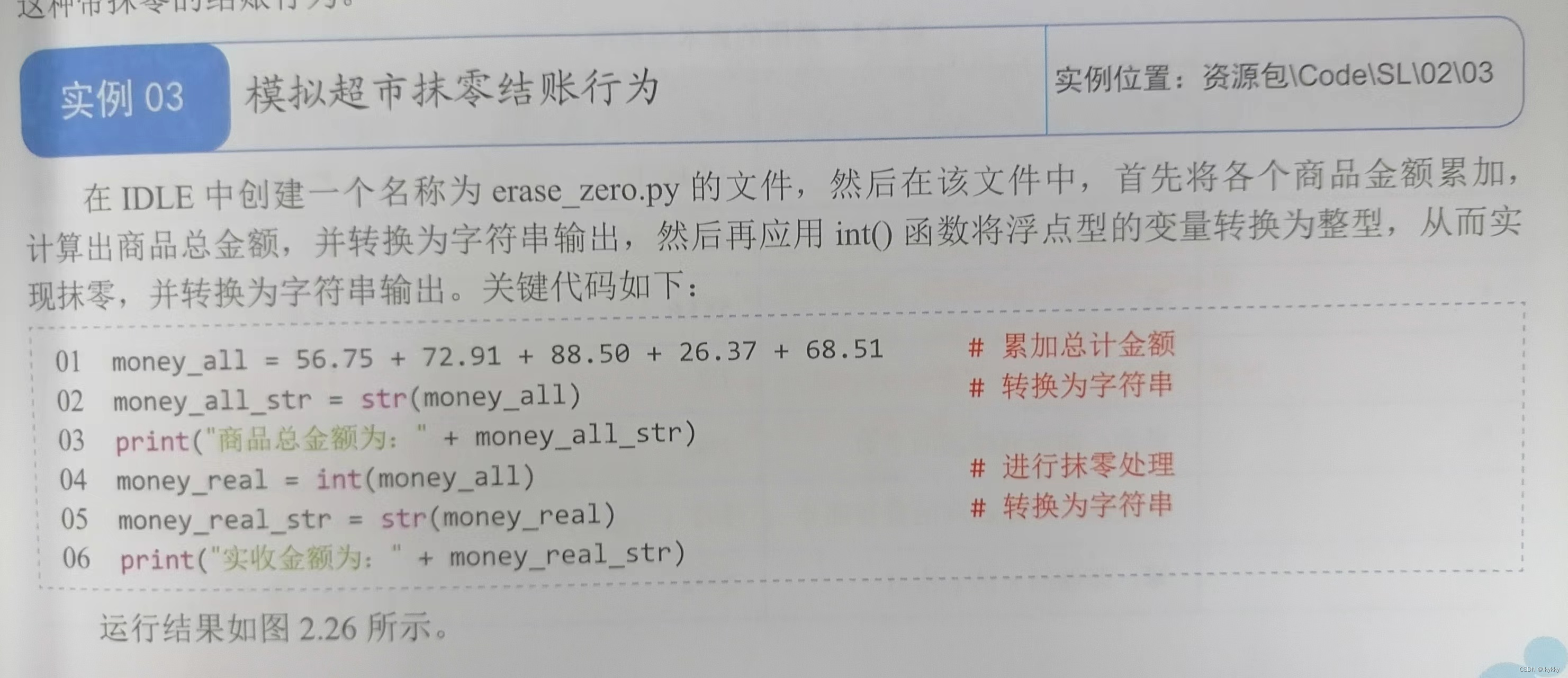``money_all=56.75+72.91+88.50+26.37+68.51money_all_str=str(money_all)print("商品总金额为："+money_all_str)money_real=int(money_all)money_real_str=str(money_real)print("实收金额为："+money_real_str)``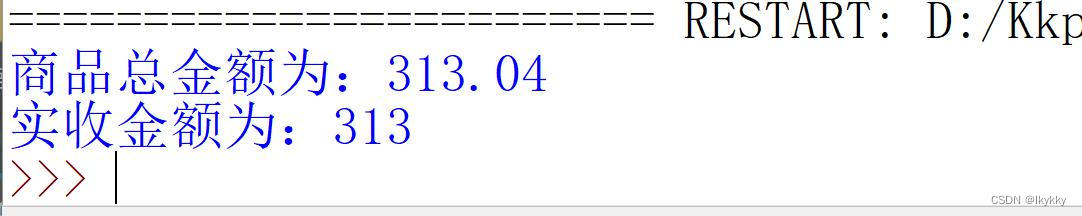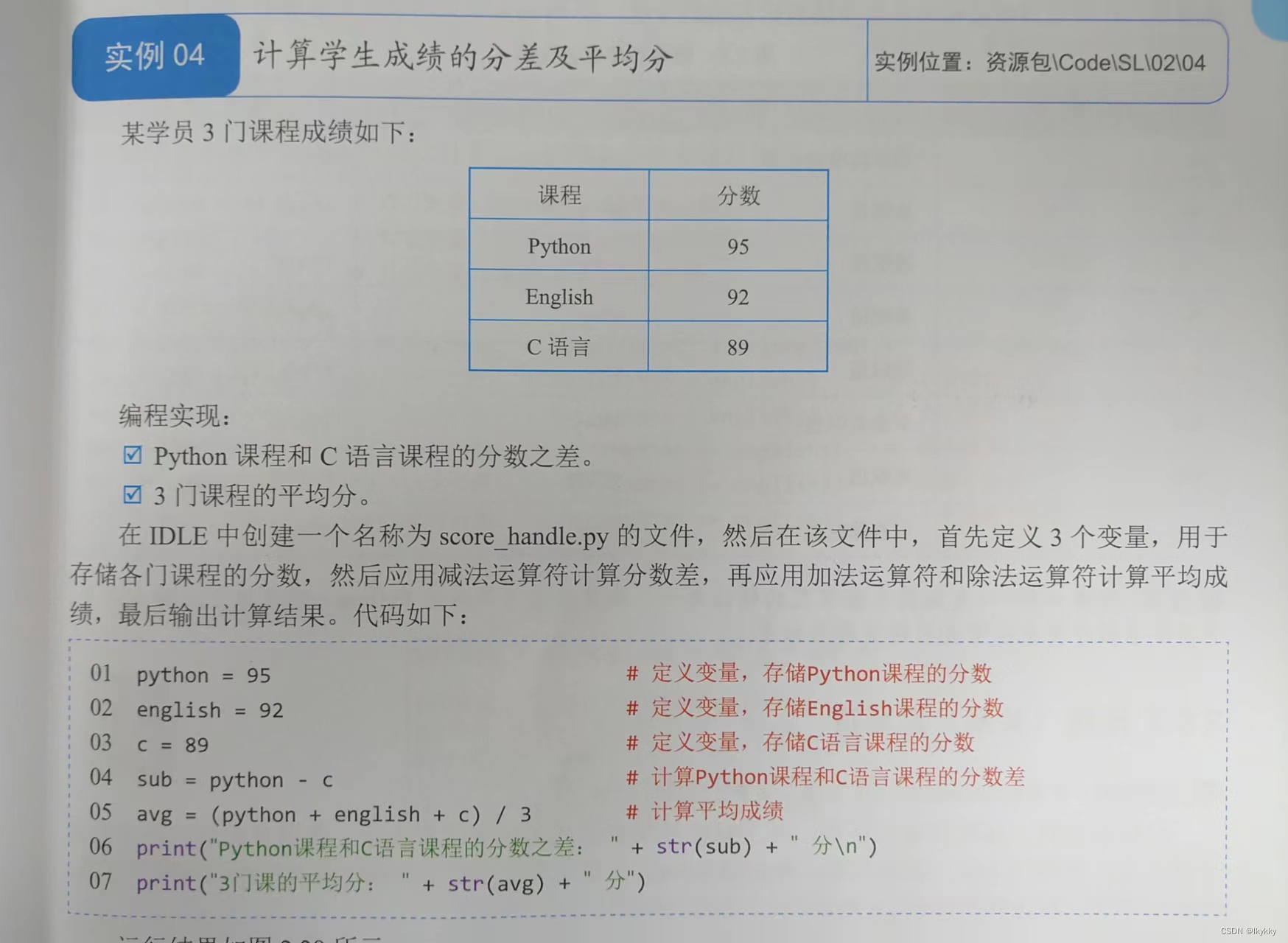``python=95english=92c=89sub=python-cavg=(python+english+c)/3print("Python课程和C语言课程的分数之差："+str(sub)+"分\n")print("3门课的平均分："+str(avg)+"分")``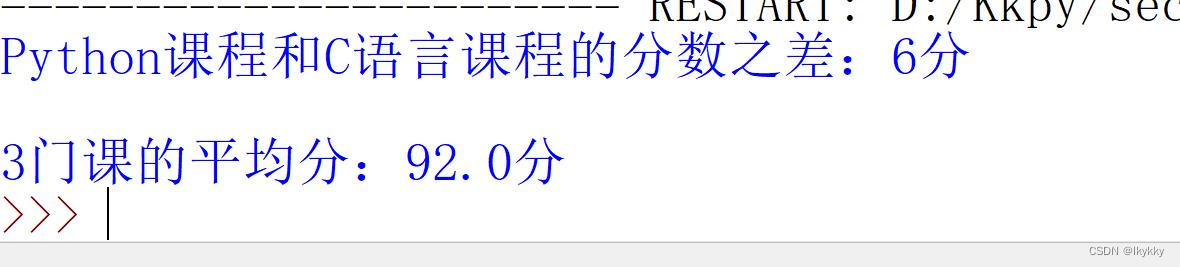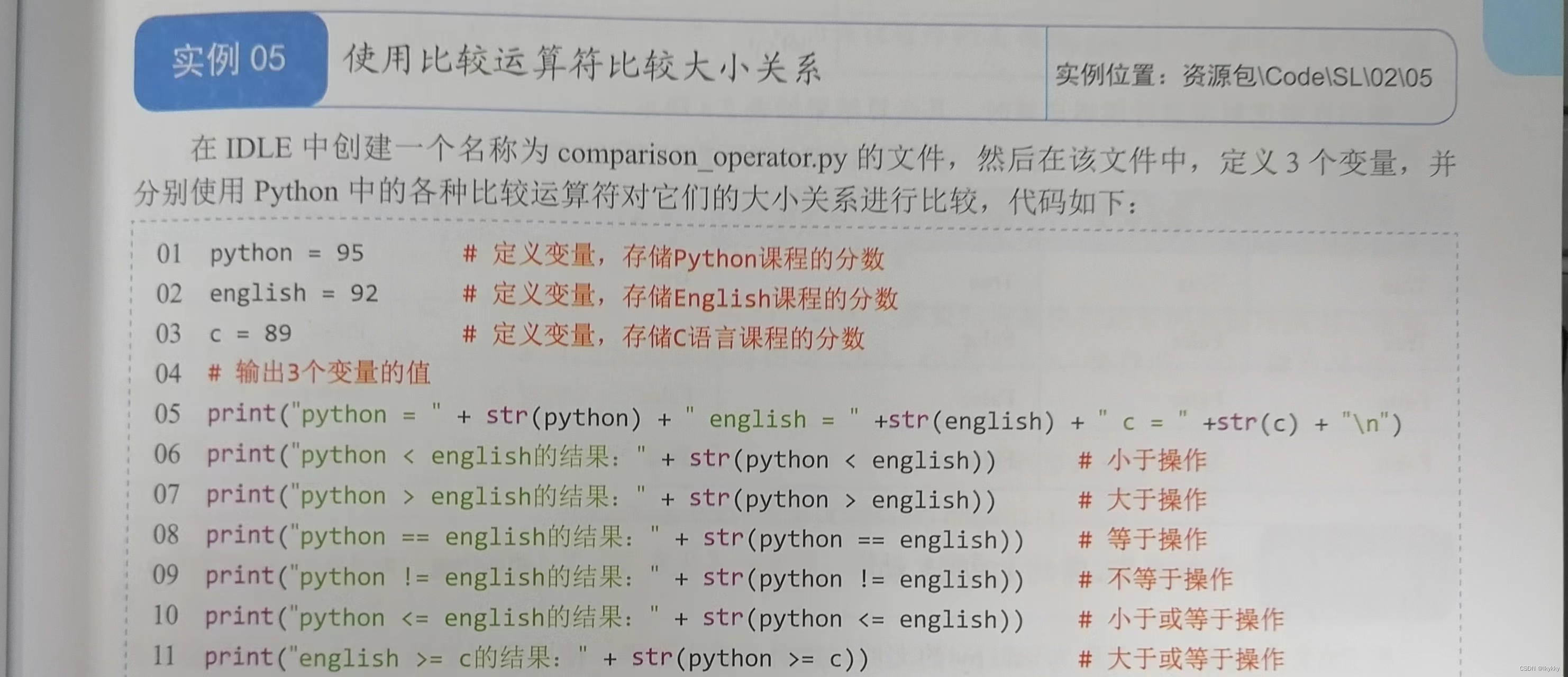``python=95       #定义变量，存储Python课程的分数english=92      #定义变量，存储English课程的分数c=89            #定义变量，存储C语言课程的分数#输出3个变量的值print("python="+str(python)+"english="+str(english)+"c="+str(c)+"\n")print("python<english的结果："+str(python<english))print("python>english的结果："+str(python>english))print("python==english的结果："+str(python==english))print("python!=english的结果："+str(python!=english))print("python<=english的结果："+str(python<=english))print("english>=c的结果："+str(python>=c)) ``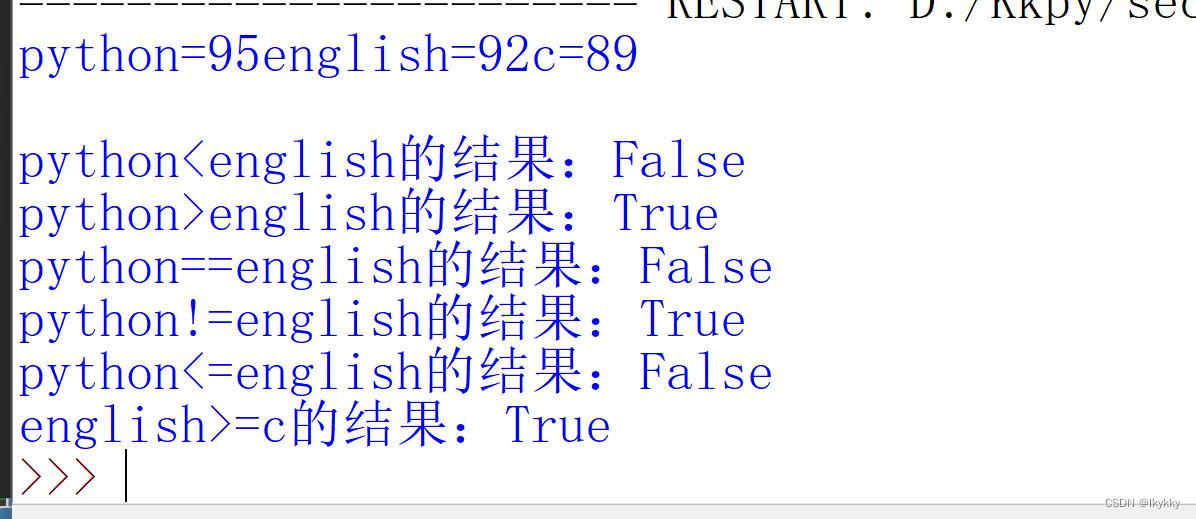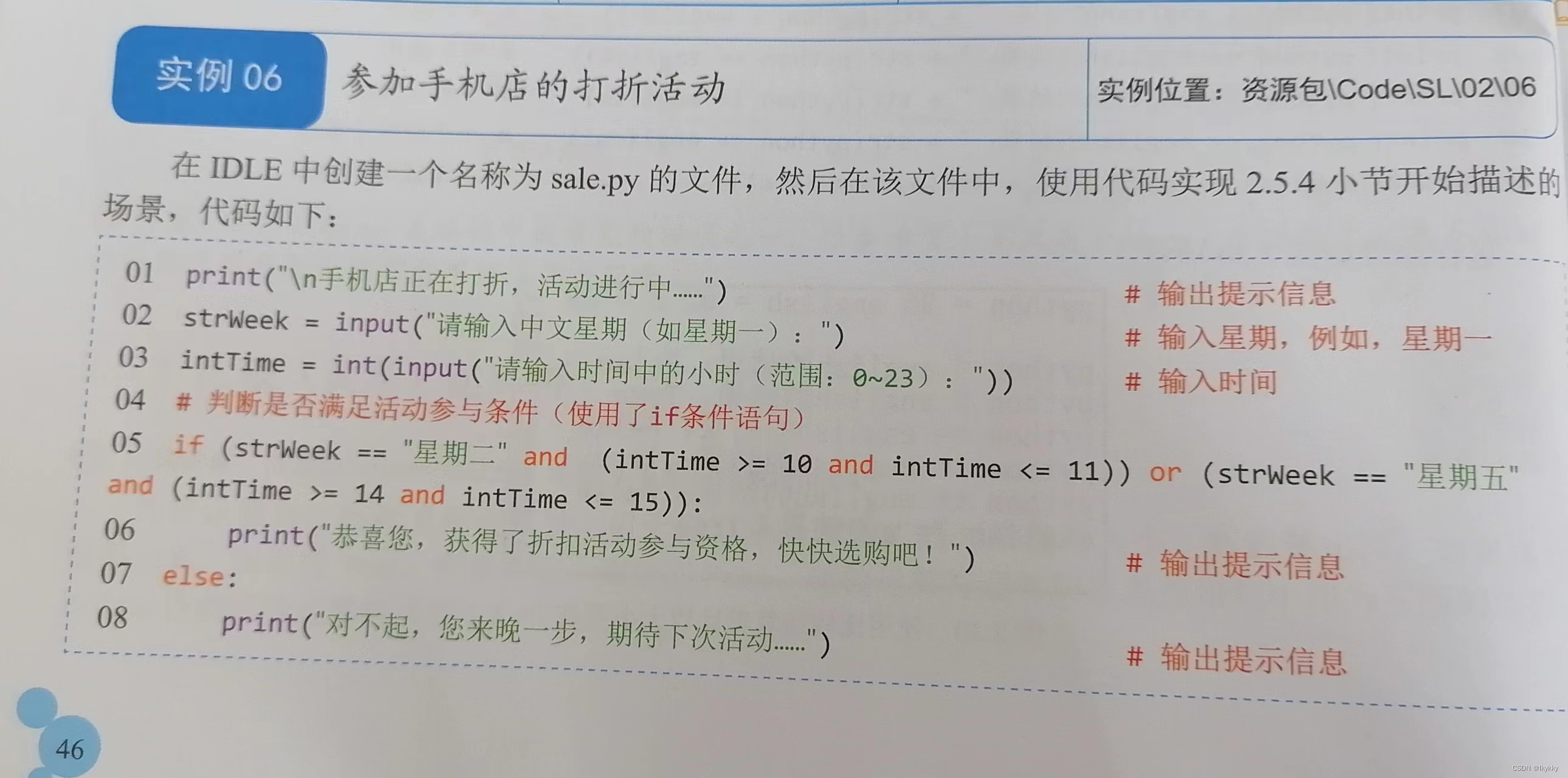``print("\n手机店正在打折，活动进行中......")strWeek=input("请输入中文星期（如星期一）：")intTime=int(input("请输入时间中的小时（范围：0~23）："))if(strWeek=="星期二"and(intTime>=10 and intTime<=11))or(strWeek=="星期五" and(intTime>=14 and intTime<=15)):    print("恭喜您，获得了折扣活动参与资格，快快选购吧！")else:    print("对不起，您来晚一步，期待下次活动...")``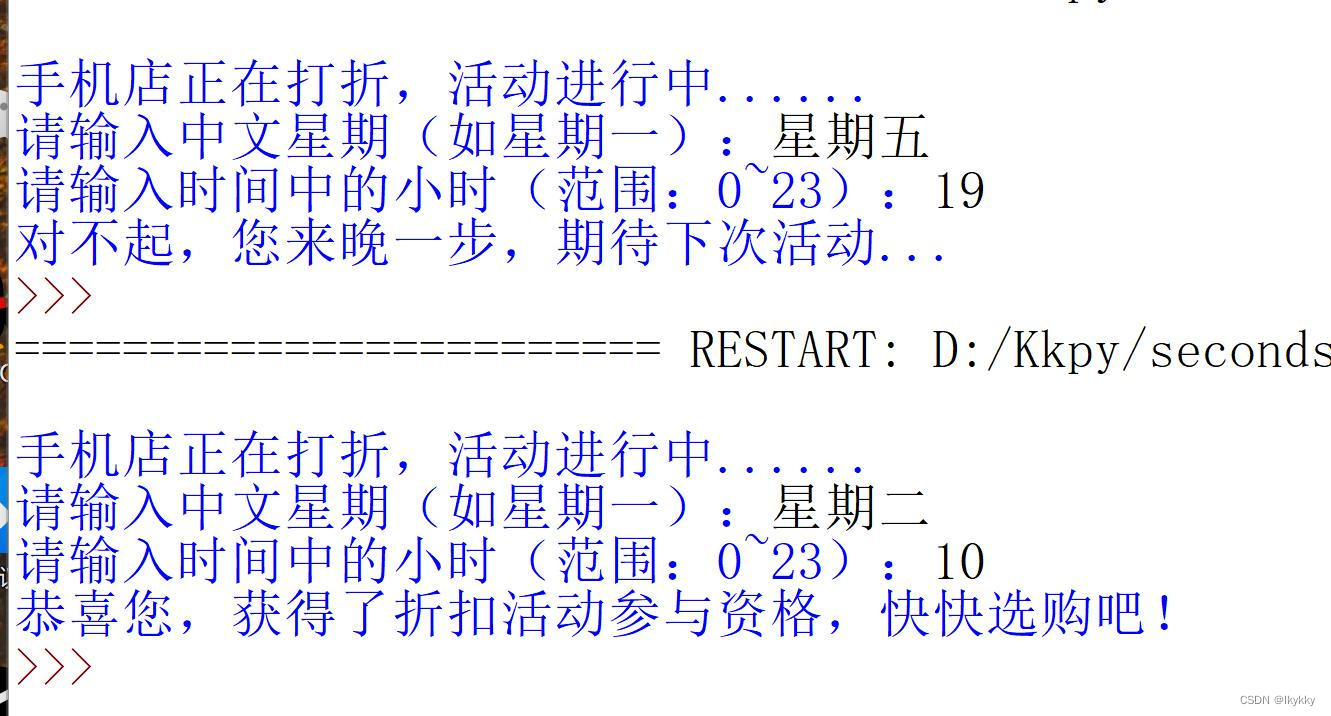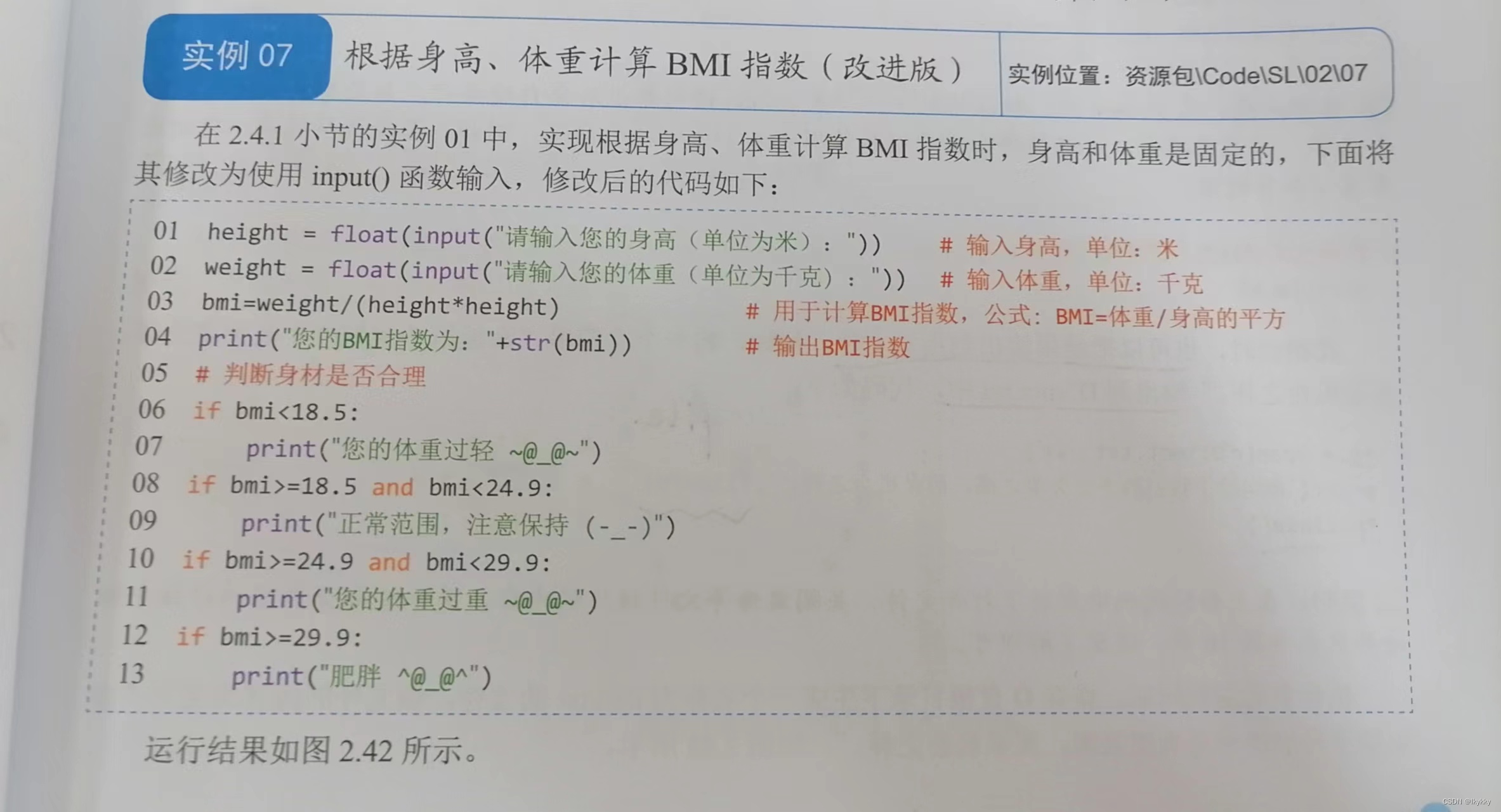``height = float(input("请输入您的身高（单位为米）："))      # 输入身高，单位：米weight = float(input("请输入您的体重（单位为千克)："))     # 输入体重，单位：千克bmi=weight/(height*height)      # 用于计算BMI指数，公式为“体重/身高的平方”print("您的BMI指数为："+str(bmi))  # 输出BMI指数# 判断身材是否合理if bmi<18.5:    print("您的体重过轻 ~@_@~")if bmi>=18.5 and bmi<24.9:    print("正常范围，注意保持 (-_-)")if bmi>=24.9 and bmi<29.9:    print("您的体重过重 ~@_@~")if bmi>=29.9:    print("肥胖 ^@_@^")``